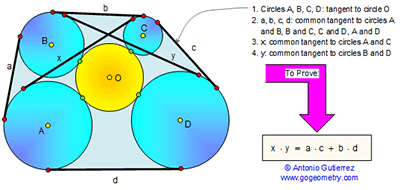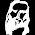## Sunday, June 14, 2015

### Geometry Problem 1121: Casey's Theorem. Generalized Ptolemy's Theorem

Level: Mathematics Education, High School, Honors Geometry, College.

Click the diagram below to enlarge it.#### 1 comment:

1.A property of circles under inversive transformation is quoted here without proof:

Let C₁ and C₂ be two circles with radius r₁ and r₂ respectively, with the length of common tangent=l.
If under an inversive transformation, they becomes another two circle C₁' and C₂',
with radius R₁ and R₂ respectively, and with length of common tangent=L.

Then L²/(R₁R₂) = l²/(r₁r₂).

***
Let the tangent points of circles A B C D with circle O are P Q R S respectively.

Choose any point X on arc AD, perform an inversion with center X.
Then circle O becomes a straight line, and circles A B C D becomes circles A' B' C' D'.

Let circles A B C D with radius r₁ r₂ r₃ r₄ and circles A' B' C' D' with radius R₁ R₂ R₃ R₄ respectively.
Let the image of the tangent points are P' Q' R' S'.

Then by Euler's theorem (similar to Ptolemy's theorem),
P'Q'×R'S' + P'S'×Q'R' = P'R'×Q'S'
P'Q'/√(R₁R₂)×R'S'/√(R₃R₄) + P'S'/√(R₁R₄)×Q'R'/√(R₂R₃) = P'R'/√(R₁R₃)×Q'S'/√(R₂R₄)

By the stated property,
PQ/√(r₁r₂)×RS/√(r₃r₄) + PS/√(r₁r₄)×QR/√(r₂r₃) = PR/√(r₁r₃)×QS/√(r₂r₄)

Hence,
PQ×RS + PS×QR = PR×QS
a×c + b×d = x×y